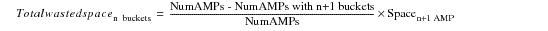# The Problem of Skew - Teradata Database

Product
Release Number
15.10
Language
English (United States)
Last Update
2018-10-06
Product Category
Software

### The Problem of Skew

Each node in a Teradata system has a set of five database hash maps, a NoPI hash map, and an Open PDE hash map, making a total of 7 hash maps. Each of these hash maps performs a different function. Teradata Database hash maps have either 65,536 or 1,048,575 entries, one for each hash bucket (see “Number of Hash Buckets Per System” on page 177). This number was carefully selected to balance the requirement that the hash maps be memory‑resident with the need to have enough buckets to avoid the skewing problems seen when an uneven number of buckets are assigned to each AMP. Unless the number of AMPs in the system divides evenly into 65,536 or 1,048,575, some skewing is unavoidable.

As an example of how a relatively small number of hash buckets quickly leads to significant skew, consider the following case. Suppose there were an imaginary Teradata system that had only 3,643 hash buckets rather than 65,536 hash buckets. Now suppose this was a large system having 200 AMPs. The result of having so few hash buckets is that some AMPs have 18 buckets and others have 19. This might seem like a small difference, but a quick computation tells a different story. The distribution of hash buckets maintained by this system guarantees a 5.26% skew of rows across the AMPs as indicated by the following calculation.The following table shows how the number of hash buckets per AMP varies with the number of AMPs in the configuration. Note that configurations with a number of AMPs that does not divide evenly into 65,536 spread the remainder buckets among the AMPs as evenly as possible. Increasing the number of hash buckets to 1,048,575 permits a still more even distribution of buckets among the AMPs.

 Number of AMPs in Configuration Number of Hash Buckets/AMP Number of AMPs With This Many Hash Buckets Percent Skew 4 16,384 4 0.0000 5 13,107 4 0.0076 13,108 1 11 5,957 2 0.0170 5,958 9 60 1,092 11 0.0900 1,093 4

Similarly, a system with 1,000 AMPs has 65 hash buckets on some AMPs and 66 hash buckets on others. In this particular case, the AMPs having 66 hash buckets also perform 1.5% more work than those with 65 hash buckets, a skew percentage of 1.515. The work per AMP imbalance increases as a function of the number of AMPs in the system for those cases where 65,536 is not evenly divisible by the total number of AMPs.

There is no calculation to determine when a system needs to convert from 16-bit hash buckets to 20-bit hash buckets, but from the figures in the following table, you can see that a system with 1,000 or more AMPs should seriously consider converting to 1,048,575 hash buckets. The table indicates how a system with 65,536 hash buckets affects the skew of row distribution for tables as a function of the number of AMPs on the system.

 Number of AMPs Low Number of Hash Buckets Per AMP High Number of Hash Buckets Per AMP Imbalance Percentage 100 655 656 0.15 200 327 328 0.31 300 218 219 0.46 400 163 164 0.61 500 131 132 0.76 750 87 88 1.14 1,000 65 66 1.52 2,000 32 33 3.03 3,000 21 22 4.55 4,000 16 17 5.88 5,000 13 14 7.14 6,000 10 11 9.09 7,000 9 10 10.00 8,000 8 9 11.11 9,000 7 8 12.50 10,000 6 7 14.29

Note that there is also a relationship between the number of hash buckets on a system and the percentage of disk space that is wasted on AMPs that have fewer hash buckets than others. This is referred to as the hash imbalance problem, where some AMPs own n hash buckets for a table, while others own n-1. The one bucket difference translates into wasted disk storage.

This relationship can be expressed as a percentage of wasted disk space as follows.where:

 Equation element … Specifies the … Total wasted spacen buckets percentage of total wasted space on AMPs that control n hash buckets. There are N-m such AMPs on any system, where N is the number of AMPs on the system and m is the number of AMPs on the system having n+1 hash buckets. NumAMPs number of AMPs in the system. NumAMPs with n+1 buckets number of AMPs in the system that own n+1 hash buckets. Spacen+1 AMP space occupied by one hash bucket on an AMP that owns n+1 hash buckets. This value is determined using the following equation.where n +1 is number of hash buckets owned by an AMP with n+1 hash buckets.

The percent wasted disk space for 65,536 versus 1,048,576 hash buckets for a given number of system AMPs decreases greatly for systems having the larger number of hash buckets.

An additional advantage of a larger number of hash buckets is the finer granularity it provides for rowhash‑level locking (see SQL Request and Transaction Processing for details about locking levels), which both improves the performance of primary index retrievals and decreases the probability of rowhash‑level locking conflicts. This is particularly true for very large tables.